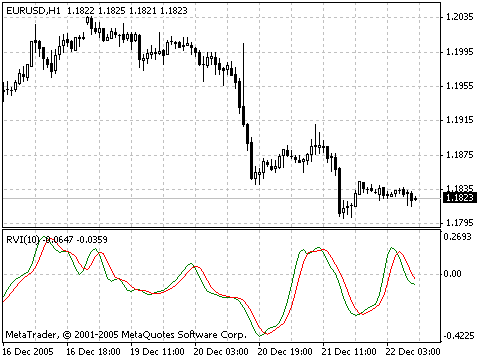Interesting script?
So post a link to it -
let others appraise it
You liked the script? Try it in the MetaTrader 5 terminal# Relative Vigor Index (RVI) - indicator for MetaTrader 4

Views:
35756
Rating:
Published:
2005.12.22 13:36
Updated:
2016.11.22 07:32

The main point of Relative Vigor Index Indicator (RVI) is that on the bull market the closing price is, as a rule, higher, than the opening price.

It is the other way round on the bear market. So the idea behind Relative Vigor Index is that the vigor, or energy, of the move is thus established by where the prices end up at the close. To normalize the index to the daily trading range, divide the change of price by the maximum range of prices for the day. To make a more smooth calculation, one uses a symmetrically weighted moving average of the differences of the closing and openings prices, as well as maximum and minimum prices of the bar.

The best period at calculation of the indicator is considered 10. To avoid probable ambiguity one needs to construct a signal line, which is a symmetrically weighted moving averageof Relative Vigor Index values. The concurrence of lines serves as a signal to buy or to sell.

Calculation

VALUE1 = ((CLOSE - OPEN) + 2 * (CLOSE (1)) – OPEN (1)) + 2*(CLOSE (2) – OPEN (2)) + (CLOSE (3) – OPEN (3))) / 6

VALUE2 = ((HIGH - LOW) + 2 * (HIGH (1) – LOW (1)) + 2*(HIGH (2)- LOW (2)) + (HIGH (3) – LOW (3))) / 6

NUM = SUM (VALUE1, N)

DENUM = SUM (VALUE2, N)

RVI = NUM / DENUM

RVISig = (RVI + 2 * RVI (1) + 2 * RVI (2) + RVI (3)) / 6

where:

• OPEN — is the opening price;
• HIGH — is the maximum price;
• LOW — is the minimum price;
• CLOSE — is the closing price;
• VALUE1 — symmetrically weighted moving average of the differences of the closing and openings prices;
• VALUE2 — symmetrically weighted moving average of the differences of the maximum and minimum prices;
• NUM — amount N importances of VALUE1;
• DENUM —amount N importances of VALUE2;
• RVI — value of the Relative Vigor Index indicator for the current bar;
• RVISig — value of the RVI signal line for the current bar;
• N — period of the smoothing.Full description of Relative Vigor Index is available in the Technical analysis: Relative Vigor Index

Translated from Russian by MetaQuotes Software Corp.
Original code: https://www.mql5.com/ru/code/8035Money Flow Index (MFI)

Money Flow Index (MFI) is the indicator, which indicates the rate at which money is invested into a security and then withdrawn from it.Standard Deviation (StdDev)

The Standard Deviation Indicator (StdDev) measures the market volatility.DailyPivotPoints

The DailyPivot Points indicator helps to get the idea of the future market movements, unlike other tools that are usually behind the market.Sell-Buy Alert

The indicator displays Buy/Sell arrows as the MACD or RVI indicators are changing.